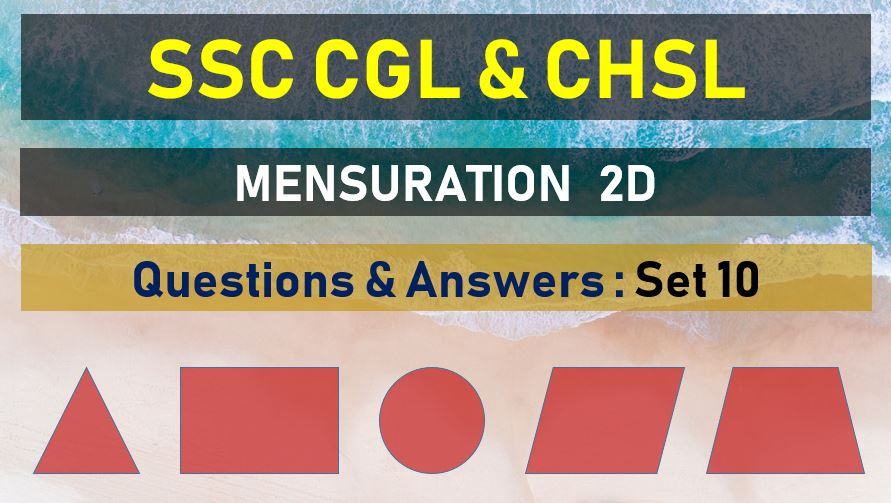Friday, May 7, 2021
Home > mensuration > SSC CHSL Area and Perimeter Questions Answers Set 10

# SSC CHSL Area and Perimeter Questions Answers Set 10SSC Mensurations 2D
Area and Perimeter Questions Sets
Set 1Set 2Set 3Set 4Set 5
Set 6Set 7Set 8Set 9Set 10

SSC CHSL Area and Perimeter Questions Answers Set 10: Ques No 1

The circumference of a circle is 11 cm and the angle of a sector of the circle is 60°. The area of the sector is (use π =22/7)

Options:

A. 77/48 cm2

B. 125/48 cm2

C. 75/48 cm2

D. 123/48 cm2

Solution:

SSC CHSL Area and Perimeter Questions Answers Set 10: Ques No 2

If the difference between areas of the circumcircle and the incircle of an equilateral triangle is 44 cm2, then the area of the triangle is ( Take π =22/7)

Options:

A. 28 cm2

B. 7√3 cm2

C. 14√3 cm2

D. 21 cm2

Solution:

SSC CHSL Area and Perimeter Questions Answers Set 10: Ques No 3

If the area of a circle inscribed in a square is 9 π cm2, then the area of the square is

Options:

A. 24 cm2

B. 30 cm2

C. 36 cm2

D. 81 cm2

Solution:

SSC CHSL Area and Perimeter Questions Answers Set 10: Ques No 4

The sides of a triangle are 6 cm, 8 cm and 10 cm. The area of the greatest square that can be inscribed in it, is

Options:

A. 18 cm2

B. 15 cm2

C. 2304/49 cm2

D. 576/49 cm2

Solution:

SSC CHSL Area and Perimeter Questions Answers Set 10: Ques No 5

The length of a side of an equilateral triangle is 8 cm. The area of region lying between the circumcircle and the incircle of the triangle is ( use π =22/7)

Options:

A. 351/7 cm2

B. 352/7 cm2

C. 526/7 cm2

D. 527/7 cm2

Solution:

SSC CHSL Area and Perimeter Questions Answer Set 10: Ques No 6

A wire, when bent in the form of a square, encloses a region having area 121 cm2. If the same wire is bent into the form of a circle , then the area of the circle is (use π =22/7)

Options:

A. 144 cm2

B. 180 cm2

C. 154 cm2

D. 176 cm2

Solution:

SSC CHSL Area and Perimeter Questions Answer Set 10: Ques No 7

If the perimeter of a semicircular field is 36 m. Find its radius (use π =22/7)

Options:

A. 7 cm

B. 8 cm

C. 14 cm

D. 16 cm

Solution:

SSC CHSL Area and Perimeter Questions Answer Set 10: Ques No 8

The perimeter (in meters) of a semicircle is numerically equal to its area ( in square meters). The length of its diameter is (use π =22/7)

Options:

A. 36/11 m

B. 61/11 m

C. 72/11 m

D. 68/11 m

Solution:

SSC CHSL Area and Perimeter Questions Answer Set 10: Ques No 9

One acute angle of a right angled triangle is double the other. If the length of its hypotenuse is 10 cm, then its area is

Options:

A. 25/2(√3) cm2

B. 25 cm2

C. 25√3cm2

D. 75/2 cm2

Solution:

SSC CHSL Area and Perimeter Questions Answer Set 10: Ques No 10

If a triangle with base 8 cm has the same area as a circle with radius 8 cm, then the corresponding altitude (in cm) of the triangle is

Options:

A. 12 π

B. 20 π

C. 16 π

D. 32 π